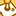# New to Qlik Sense

If you’re new to Qlik Sense, start with this Discussion Board and get up-to-speed quickly.

Announcements
Our next Qlik Insider session will cover new key capabilities. Join us August 11th REGISTER TODAY
cancel
Showing results for
Did you mean:Creator

## Help with showing 'Percentage of numbers in a range'?

Hello all.

My chart is successfully showing the percentage of numbers above 4 like this...

Num(Count({<Score = {">=4"}>} Score) /Count(Score),'0%')

How would I go about altering this to show percentages in a range? For instance between 3.0 and 3.99?

Jordan

1 Solution

Accepted SolutionsMaster II

Guess

Num(Count({<Score= {">=3<=3.9"}>} Score) /Count(Score),'0.0%')

4 RepliesMaster II

Num(Count({<Score = {">=4"}>} Score) /Count(Score),'0.0%')

Your expression would only show values which are greater or equal to 4.

If you want 3 as well then use

Num(Count({<Score = {">=3"}>} Score) /Count(Score),'0.0%')

or if you want all %'s

Num(Count({<Score = {">=0"}>} Score) /Count(Score),'0.0%')Creator
Author

Hi, thanks for the response.

Yes I know that the expression only shows value which are greater than or equal to 4.

I was wondering how I would write this expression to show a range of values. Specifically between 3.0 and 3.9.Master II

Guess

Num(Count({<Score= {">=3<=3.9"}>} Score) /Count(Score),'0.0%')Creator
Author

That appears to have worked. Thanks for the help my friend.Tags
Community Browser